# Class 10 Maths NCERT solutions for Polynomials Exercise 2.1

## Class 10 Maths NCERT solutions Chapter 2 Exercise 2.1

In this page we have Class 10 Maths NCERT solutions for Polynomials for Exercise 2.1 on page 28 . Hope you like them and do not forget to like , social_share and comment at the end of the page.
Question 1
The graphs of y = p(x) are given below, for some polynomials p(x). Find the
number of zeroes of p(x), in each case.
(i) and (ii)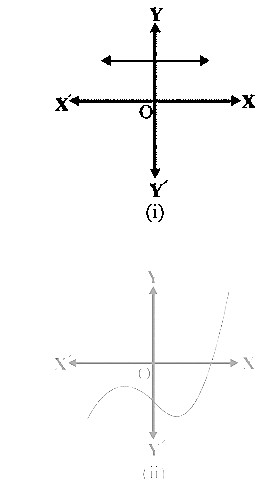(iii)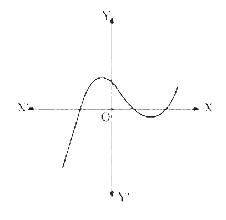(iv)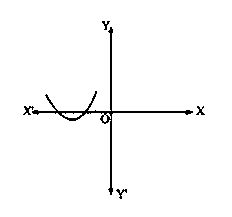(v)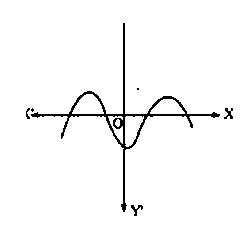(vi)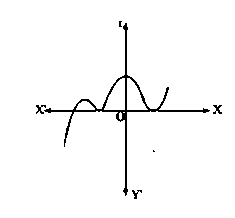Solution:
The zero’s of the polynomial are the points  where the graph meet x axis in the Cartesian plane. If the graph does not meet x axis ,then the polynomial does not have any zero’s.
1.  Since it does not intersect x-axis ,it does not have any zero’s
2. Since it intersect x-axis once ,it has one zero
3. Since it intersect the x-axis thrice,it has three zero’s
4. Since it intersect the x-axis two,it has two zero’s
5. Since it intersect the x-axis four times,it has four zero’s
6. Since it intersect the x-axis thrice,it has three zero’s

## Summary

1. Class 10 Maths NCERT solutions for Polynomials Exercise 2.1 has been prepared by Expert with utmost care. If you find any mistake.Please do provide feedback on mail. You can download the solutions as PDF in the below Link also
2. This chapter 2 has total 4 Exercise 2.1 ,2.2,2.3 and 2.4. This is the First exercise in the chapter.You can explore previous exercise of this chapter by clicking the link belowGo back to Class 10 Main Page using below links

### Practice Question

Question 1 What is $1 - \sqrt {3}$ ?
A) Non terminating repeating
B) Non terminating non repeating
C) Terminating
D) None of the above
Question 2 The volume of the largest right circular cone that can be cut out from a cube of edge 4.2 cm is?
A) 19.4 cm3
B) 12 cm3
C) 78.6 cm3
D) 58.2 cm3
Question 3 The sum of the first three terms of an AP is 33. If the product of the first and the third term exceeds the second term by 29, the AP is ?
A) 2 ,21,11
B) 1,10,19
C) -1 ,8,17
D) 2 ,11,20